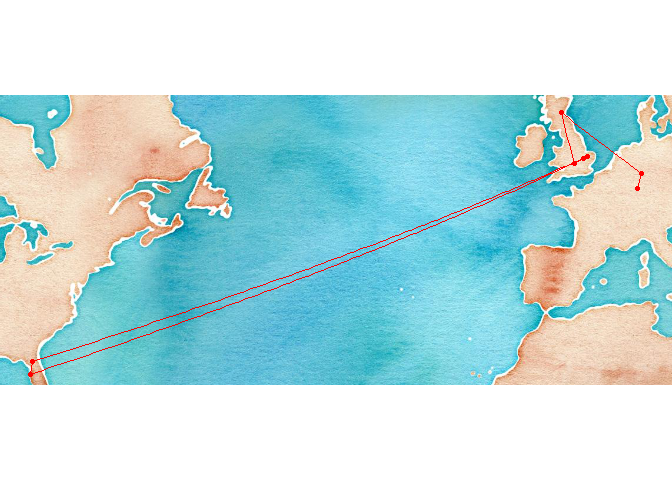# Dr Philipp Boersch‑Supan

Quantitative Ecologist

## Mapping one's academic career path

Yesterday I came across Gordon Pennycook’s tweet about moving in academia:

So given that there’s a transatlantic move coming up for me to take up my first permanent research position at the British Trust for Ornithology, I thought, why not figure this out quickly using R.

``````library(ggmap)# for geocoding and plotting
library(geosphere)# for distance calculations
library(knitr)# for making a nice table
``````

I used `ggmap::geocode` to look up the coordinates of each station on my academic career path:

``````academic_places <- geocode(c(home = "Neustadt an der Weinstrasse",
undergrad = "Marburg an der Lahn",
masters_phd = "St Andrews, Fife",
phd = "Oxford, Oxfordshire",
postdoc1 = "Cambridge, UK",
postdoc2a = "Tampa, FL",
postdoc2b = "Gainesville, FL",
job = "Thetford"),
source = "dsk")
``````

A quick plot to sanity check the locations

``````#make a map
qmplot(lon, lat, data = academic_places, maptype = "watercolor", color = I("red")) + geom_path(color = "red")
``````I then used the `geosphere` package to calculate sequential distance between stations

``````#calculate distances

#transform units
distances_km <- distances_m/1000
distances_mi <- distances_m/1609
``````

And lastly, I made a table to sum up everything.

``````#make a table
kable(data.frame(stage = c(academic_places[-1,1], "Total"),
distance_km = round(c(distances_km, sum(distances_km))),
distance_mi = round(c(distances_mi, sum(distances_mi)))))
``````
stage distance_km distance_mi